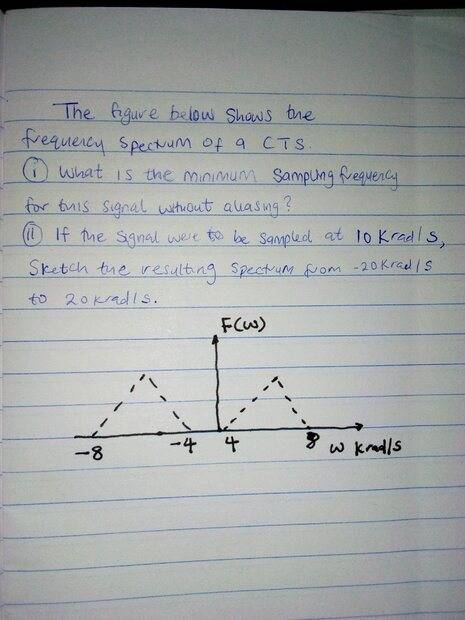# Sampling frequency to use with this signal to avoid aliasing

• Engineering
Homework Statement:
The figure below shows the frequency spectrum of a CTS.
Relevant Equations:
The sampling frequency without aliasing. I am posting this here because I don't even know how to approach the question. Is there a way to approach this kind of question. Thank you.i am

## Answers and Replies

FactChecker
Science Advisor
Gold Member
Are you familiar with the Nyquist frequency and the Nyquist rate?

Are you familiar with the Nyquist frequency and the Nyquist rate?
Yes. I am familiar till the part that the maximum angular frequency is 8 rad/s. So using the Nyquist rate, the sampling frequency should be twice the max frequency. But I don't understand how to systematically approach this kind of question. Thank you.

FactChecker
Science Advisor
Gold Member
Do you mean that you don't know how to systematically use the Nyquist rate or what?
You have answered the first question.
For the second question, assume point-by-point that anything below 1/2 the sampling frequency is not aliased and that everything above it is aliased to a lower frequency. What would the aliased spectrum graph look like?

PS. The simple Nyquist sample rate theorem (with perfect suppression of aliasing) assumes that the sample is taken for an infinite time. There is a more complicated version for a limited sample time, but it is rarely used and I can not find a reference for it.

Last edited:
bobob
Gold Member
Yes. I am familiar till the part that the maximum angular frequency is 8 rad/s. So using the Nyquist rate, the sampling frequency should be twice the max frequency. But I don't understand how to systematically approach this kind of question. Thank you.
Sort of. You have a triangle wave, so theoretically, your sampling frequency needs to be infinite since there are frequency components going out to infinity. The way to approach it is to do a fourier decomposition of the triangle wave and decide how well you need the reconstruction to resemble the triangle wave. Sampling at twice the frequency of the triangle wave will result in reconstructing a sine wave with the frequency of the triangle wave (ignoring aliasing). You need to sample at twice the highest frequency in the fourier series you want to use to reconstruct the reconstruction of the triangle wave with the precision you require. Since any "real" triangle wave will have a finite bandwidth and not be a perfect triangle wave, you might start there.

FactChecker
Science Advisor
Gold Member
Sort of. You have a triangle wave, so theoretically, your sampling frequency needs to be infinite since there are frequency components going out to infinity.
The figure shown is the frequency spectrum, not the wave. So it is much simpler.

bobob
Gold Member
The figure shown is the frequency spectrum, not the wave. So it is much simpler.
Oops... My mistake for not looking at the figure more closely...